﻿ Rheology Properties of Castor Oil: Temperature and Shear Rate-dependence of Castor Oil Shear Stress
Journal of Material Sciences & Engineering
All submissions of the EM system will be redirected to Online Manuscript Submission System. Authors are requested to submit articles directly to Online Manuscript Submission System of respective journal.
• Research Article
• J Material Sci Eng 2015, Vol 5(1): 220
• DOI: 10.4172/2169-0022.1000220

# Rheology Properties of Castor Oil: Temperature and Shear Rate-dependence of Castor Oil Shear Stress

Abdelraziq IR* and Nierat TH
Physics Department, An-Najah National University, Nablus, Palestine
*Corresponding Author: Abdelraziq IR, Physics Department, An-Najah National University, Nablus, Palestine, Tel: 092345113, Email: [email protected]

Received Date: Nov 18, 2015 / Accepted Date: Dec 05, 2015 / Published Date: Dec 15, 2015

### Abstract

The dynamic viscosity of castor oil was measured as a function of shear rate at different temperature ranged from 1.7°C to 62°C. In this study, shear stress and the dynamic viscosity as a function of temperature of castor oil decrease with increasing temperatures. Three and multi-constant formulas were proposed to obtain more suitable prediction of temperature dependence of shear stress and dynamic viscosity of castor oil. The best AAD% was calculated using our proposed formulas to be 0.03%. This work shows that the behavior of castor oil at the temperature ranged from 1.7°C to 62°C is Newtonian behavior by fitting the model of power law.

Keywords: Castor oil; Viscosity; Shear stress; Temperature; Formulas

#### Introduction

The rheology is the science of deformation and the study of the manner in which materials respond to applied stress or strain [1,2]. There are several studies that proposed alternative equations to describe the dynamic viscosity of liquids as a function of temperature. Two constant, three constant and multi-constant forms were expressed to describe the viscosity of vegetable oil as a function of temperature. Stanciu in his study, proposed four relationships of dynamic viscosity temperature dependence for vegetable oils. The purpose of his study was to find a polynomial or exponential dependence between temperature and dynamic viscosity of vegetable oil . Giap derived an equation to replace the well-known Arrhenius-type relationship, his model was tested by using six vegetable oils . Multi-constant forms to represent the liquid viscosity as a function of temperature was proposed by Thorpe and another by Daubert [5,6]. Three-constant forms also were proposed by De Guzman and by Vogel [7,8]. The modified versions of the Andrade equation were used by Abramovic to describe the effect of temperature on dynamic viscosities for a number of vegetable oils. In addition, new forms to describe the effect of temperature on viscosity were suggested by Abramovic .

The flow behavior of liquids (Newtonian or non-Newtonian) was explained by Zhou. In his paper, the shear rate and temperature dependencies of viscosity of alumina nano-fluids were investigated experimentally . The dynamic viscosity and shear stress, as a function of shear rate of chitosan dissolved in weakly acid solutions were studied by Esam. His results showed that shear thinning behavior is as pseudoplastic (non- Newtonian behavior) at temperature range from 20°C to 50°C, but it is showed more remarkable at lower temperature . In Akhtar’s study, Newtonian or Non-Newtonian behaviors of different oil samples (olive, almond, coconut, castor, sesame, cottonseed, sunflower and paraffin) were investigated. All the samples of oil investigated were found to possess Newtonian behavior with little deviation in olive and coconut oils . Giap in his study, few food grade vegetable oils were subjected to viscometer measurements of viscosity at shear rate and temperature ranged from 3 to 100 rpm, and 40°C to 100°C, respectively. Results have shown that vegetable oils behaved as pseudoplastic . Rheological properties of different samples of olive oils were obtained in a wide range of temperature by Ashrafi . The dynamic viscosity of three commercial coconut fats was measured as a function of shear rate. The flow behavior of coconut fats was explained according to Newton’s equation. The dynamic viscosity of coconut fats at different temperatures was used to calculate the flow activation energy . Fasina in his study, the viscosities and specific heat capacities of twelve vegetable oils were experimentally determined as a function of temperature (35°C to 180° C) . The temperature dependent rheological behavior of un-used (soybean, sunflower, olive, rapeseed, corn, rice and the mixtures soybean + olive and sunflower + olive) and used vegetable cooking oils was evaluated by Santos . The viscosities of the three oil types (soybean oil, Sunflower oil and Canola oil) were investigated as a function of the shear rate and also shear stress as a function of shear rate at temperatures ranging from 10°C to 80°C . In Stefanescu’s paper the variation of the dynamic viscosity with the shear stress for to non-additive vegetable oils rape seed and soya bean . The viscosity as a function of temperature from 24°C to 110°C was measured by Noureddini for a number of vegetable oils (crambe, rapeseed, corn, soybean, milk-weed coconut, and lesquerella) .

The aim of this study is to evaluate the rheological properties of castor oil. The dependence of dynamic viscosity of castor oil on temperature, shear rate and shear stress will be studied. The relationship between the dynamic viscosity of castor oil with temperature shear rate and shear stress will be found by fitting equations. The flow behavior of castor oil will be explained whether it is Newtonian or non-Newtonian.

#### Theory

Viscosity is a measure of the resistance to flow or shear. There are two types of viscosity:

Dynamic viscosity (η)

Dynamic viscosity which is defined as the ratio of shear stress (force over cross section area) to the rate of deformation (the difference of velocity over a sheared distance) and it is presented as: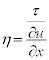(1)

Where, η is the dynamic viscosity in Pascal-second (Pa.s); τ is shear stress (N/m2); and,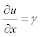is rate of deformation or velocity gradient or better known as shear rate (1/s).

Kinematic viscosity (ν)

The Kinematic viscosity requires knowledge of mass density of the liquid (ρ) at that temperature and pressure. It is defined as: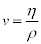(2)

Where, ν is kinematic viscosity in centistokes (cSt), ρ is in g/cm3 . Fluids such as water and benzene are Newtonian. This means that a plot of shear stress versus shear rate at a given temperature is a straight line with a constant slope that is independent of the shear rate. This slope is the viscosity of the fluid. Also, the plot passes through the origin, that is, the shear rate is zero when the shear stress is zero .

Any fluid that does not obey the Newtonian relationship between the shear stress and shear rate is called non-Newtonian. In this case, the slope of the shear stress versus shear rate curve will not be constant as one changes the shear rate. When the viscosity decreases with increasing shear rate, one calls the fluid shear-thinning. In the opposite case where the viscosity increases as the fluid is subjected to a higher shear rate, the fluid is called shear-thickening. Shear-thinning fluids also are called pseudoplastic fluids. The behavior of liquids is usually evaluated by applying the power law model of the form: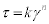(3)

Where τ is shear stress (N/m2), γ is shear rate (1/s). K is the consistency coefficient (N/m2.sn) and n is the flow behavior index .

The flow index of Eq.(3) will be calculated. If the flow index (n) of a liquid is 1, then it is Newtonian and if the value of flow index deviated from 1 then it shows the Non-Newtonian behavior. Several studies have been carried out on the effect of temperature on dynamic viscosity of vegetable oil. Many empirical relations have been proposed to describe this temperature dependence. Simple form of Andrade equation generally used to describe the effect of temperature on dynamic viscosity given by: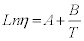(4)

Nierat used the Andrade equations of three-constant formula are represented in the following equation [23,24].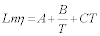(5)

Neelamegam and Abramovic also used Eq.5 in their study [9,25]. Another formula of three-constant of Andrade equation was used by Neelamegam and Abramovic, which is given by [9,25]: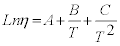(6)

Where η is the dynamic viscosity in Pa.s, T is the temperature in Kelvin. A, B and C are constants. The constants of Eq.(5) of castor oil and other oils are presented by Neelamegam .

A polynomial dependence between temperature and dynamic viscosity of vegetable oil was found by Stanciu, which are:

η = A+ BT +CT 2(7)

η = A+ BT +CT 2 + DT3 (8)

Where η is the dynamic viscosity in Pa,s and T is the temperature in Kelvin. A, B, C and D are constants .

#### Methodology

The viscosity of grad castor oil samples of crop 2012 from ALZahra factory was measured as a function of shear rate at different temperature. Shear rate and temperature ranged from 6 to 60 rpm, and 2°C to 60°C, respectively. The experimental data were fitted and the correlation constants of the best fits were estimated.

Experimental apparatus

The viscosity of castor oil samples were measured using the NDJ- 1 Rotational Viscometer with accuracy ± 5%. The spindles SP-2, SP-3 and SP-4 were operated at different speeds (6, 12, 30 and 60 rpm). Temperature was measured using Digital Prima Long Thermometer with accuracy ± 1% which measures temperature ranges from –20°C to +100°C. The Refrigerated and Heating Circulators F25-HD was used to increase and decrease the temperature of the castor oil samples to a specific temperature.

Statistical analysis

Some empirical relations were found to describe the dependence of dynamic viscosity and shear stress on temperature. The statistical analysis of the data was done by using Microsoft Excel Program. The correlation constants for the best fit were estimated. The best fit equation was chosen based on the percentage of average absolute deviation (%AAD) of the data .

#### Results and Analysis

The dependence of shear stress on shear rate

Newtonian or Non-Newtonian behavior of castor oil was investigated at different temperature ranged from 1.7°C to 62°C. Castor oil shows Newtonian behavior at the temperature ranged from 1.7°C to 62°C. Our experimental data of dynamic viscosity of castor oil was fitted using the model of power τ = kγn law. The values of flow index (n) and the consistency coefficient (K) are tabulated in Table 1. According to power law model, if the flow index (n) of a liquid is 1, then it is Newtonian and if the value of flow index deviates from 1 then it shows the Non-Newtonian behavior. Table 1 shows that castor oil has flow index value very close to 1 at the temperature ranged from 1.7°C to 62°C.

T (°C) K n SP
1.7 10.81 0.96 4
2 06.34 1.05
4 04.40 1.09
6 03.60 1.11
8 02.84 1.11
10 03.21 1.06
12 02.54 1.04
14 02.36 1.04
16 02.07 1.03
18 00.82 1.05 3
20 00.66 1.05
22 00.52 1.07
24 00.52 1.04
26 00.43 1.05
28 00.39 1.06
30 00.35 1.06
32 00.31 1.06
34 00.36 1.00
40 00.33 1.00 2
42 00.30 0.99
44 00.29 0.98
46 00.27 0.97
48 00.23 0.98
50 00.18 1.00
52 00.15 1.00
54 00.14 1.02
56 00.11 1.04
58 00.09 1.08
60 00.10 1.00
62 00.09 1.00

Table 1: Our values of flow index (n) and the consistency coefficient (K) using power law model.

Figures 1a-2b show our experimental data and our fitting curves using power law model of shear stress of castor oil as a function of shear rate.

Figure 1: (a-c) The shear stress of castor oil as function of shear rate. The solid lines are representing power law model and the points are representing our experimental data.

Figure 2: (a and b) The shear stress of castor oil as function of shear rate. The solid lines are representing power law model and the points are representing our experimental data.

The dependence of dynamic viscosity on temperature

The dynamic viscosity of castor oil as a function of temperature is plotted in Figure 3. A comparison was made between the measured experimental data of dynamic viscosity (ηexp) and the previously calculated values (ηcal). The calculated values found by three-constant formula of Andrade's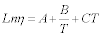. Where A, B and C are constants for castor oil (Table 2). It was found that the literature values didn’t fit our experimental data. Using Andrade's formulas, the AAD% values were found by this work to be 99.96%. As a result, Andrade's formula was not the best fit for our experimental data of dynamic viscosity of castor oil. Accordingly, a modification was introduced to Andrade's formula in order to obtain a suitable description of our experimental data of dynamic viscosity as a function of temperature. The constants of Andrade's formula were determined using the modification. Our experimental values (ηexp) and calculated values (ηcal), using the modified form of Andrade's formulas of dynamic viscosities at different temperatures is given. AAD% value of the modified form of Andrade's formulas was found to be 0.48%. The results indicate that the modified form of Andrade's formula didn’t fit exactly our experimental data. The values of the constants of the modified form of Andrade's formula are given in Table 3. These values are in disagreement with Neelamegam's values (Table 2) .

A B C Temperature range (K)
3.1924 24.8450 -0.0389 303 - 363

Table 2: The constants given by Neelamegam using Andrade's formula.

A B (K) C (K2) Temp. Range (K) AAD%
-10.5381 4393.397 -0.01584 291 - 307 0.48

Table 3: Our values of A, B and AAD% using the modified Andrade's formula of castor oil.

Figure 3: The measured values of the dynamic viscosity of castor oil as a function of temperature.

Multi-constant formulas were proposed by this work to obtain more suitable prediction of temperature dependence of dynamic viscosity of castor oil. The ηexp and ηcal were used to propose the formulas that fit our experimental data. That is, AAD% value is chosen to select the suitable prediction. It is found that our proposed formula of multiconstant to be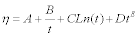which fits our experimental data of dynamic viscosity. Our calculated values of A, B, C, D, E and AAD% of the data, are given in Table 4 shows that AAD% = 0.03%; therefore, our proposed multi-constant formulas are more suitable to describe the temperature dependence of dynamic viscosity of castor oil. Figure 4 shows our experimental data and our fitting curves using our proposed multi-constant formula of dynamic viscosity of castor oil as a function of temperature.

A (cP) B (cP.°C) C (cP) D(cP/°CE) E Temp Range (°C) AAD%
-2238.88 21547.23 500.5564 60258.99 -1.60718 18 - 34 0.03

Table 4: Our values of A, B, C, D, E and AAD% using our proposed multi-constants formula.

Figure 4: The dynamic viscosity castor oil as function of temperature. The solid lines are representing our proposed multi-constant formula and the points are representing our experimental data.

The dependence of shear stress on temperature

The shear stress of castor oil as a function of temperature at 60 rpm is plotted in Figure 5. Three and multi-constant formulas were proposed by this work to obtain suitable prediction of the dependence of shear stress of castor oil on temperature. The τexp and τcal were used to propose the formulas that fit our experimental data. That is, AAD% values are chosen to select the suitable prediction.

Figure 5: The measured values of shear stress of castor oil as a function of temperature at 60 rpm.

The experimental values of the shear stress of castor oil were fitted by using our three and multi-constant formulas. Our three and multiconstant formulas are proposed to be:

τ = A+ BtC (9)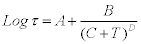(10)

Where τ is shear stress (N/m2), T is the temperature in Kelvin, t is the temperature in degrees Celsius. Our calculated values of A, B, C, D, AAD% are given in Table 5. Table 5 shows that AAD% values of three and multi-constant are- 0.03% and -0.08% respectively; therefore, our proposed three and multi-constant formula are suitable to describe the dependence of shear stress of castor oil as a function of temperature.

The formula A B C D Temp Range AAD%
Three-constant 7.214N/m2 15297.458 -1.967((N/m2)/°CC)   18 – 34°C -0.03
Multi constant 0.638 5.343KD -264.468K 5.638 291 – 307 K -0.08

Table 5: Our values of A, B, C, D and AAD% using our proposed three &multiconstants formula.

Figures 6 and 7 show our experimental data and our fitting curves using our proposed three and multi-constant formulas of shear stress of castor oil as function of temperature.

Figure 6: The shear stress of castor oil as a function of temperature. The solid lines are representing our proposed three-constant formula and the points are representing our experimental data.

Figure 7: The shear stress of castor oil as a function of temperature. The solid lines are representing our proposed multi-constant formula and the points are representing our experimental data.

#### Discussion

Our measured flow index (n) values of castor oil ranged between 0.96 - 1.11 (which are very close to 1) at the temperature that ranged from 1.7°C to 62°C. Our calculated values of the coefficient (K) of castor oil are found to be from 0.09 to 10.81. This indicates that castor oil shows Newtonian behavior at the temperature ranged from 1.7°C to 62°C. Akhtarin his study also shows the behavior of castor oil to be Newtonianin nature. Akhtar obtained the flow index of castor oil to be 0.98°C at 25°C .

Our dynamic viscosity of castor oil was measured to be 800.00 cP at 20°C, 440.00 cP at 30°C, and 325.00 cP at 40°C. Robert obtained the dynamic viscosity of castor oil to be 986.00 cP at 20°C . Robert and Neelamegam obtained the dynamic viscosity of castor oil to be 451.00 cP and 329.37 cP, at 30°C, respectively. Robert and Neelamegam obtained also 231.00 cP and 271.35 cP, at 40°C, respectively [25,26].

Our values of dynamic viscosity of castor oil at high temperatures (only) are in good agreement with Neelamegam's and Robert's values. The measured experimental results of dynamic viscosity of castor oil are compared against the previously calculated values found by Andrade's formula of three-constants which is given by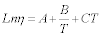. For instance, the calculated value of dynamic viscosity at 30°C was found to be 0.20 cP. Where, our measured value at 30°C is 440.00 cP. This shows significant difference between our result and the literature value. This indicates that Andrade's formulas are not the best fit to be used for dynamic viscosity of castor oil. So, Andrade's formula is modified to fit our experimental values. As a result of this modification, the constants A, B and C were determined using Andrade's formula. The calculated dynamic viscosity using the modified form of Andrade's formulas at 30°C is found to be 432.84 cP, which indicates that Andrade's modified formula does not fit exactly our experimental data. The multi-constant equation given by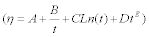is proposed to obtain more suitable prediction of temperature dependence of dynamic viscosity of castor oil. The constants of our proposed formula were estimated to give the best fit. The results of shear stress of castor oil in this study indicate that the shear stress of castor oil decreases as a function of temperature increases.

#### References

Citation: Abdelraziq IR, Nierat TH (2015) Rheology Properties of Castor Oil: Temperature and Shear Rate-dependence of Castor Oil Shear Stress. J Material Sci Eng 5: 220. Doi: 10.4172/2169-0022.1000220

Copyright: © 2015 Abdelraziq IR, et al. This is an open-access article distributed under the terms of the Creative Commons Attribution License, which permits unrestricted use, distribution, and reproduction in any medium, provided the original author and source are credited.

Select your language of interest to view the total content in your interested language

##### Recommended Journals
Viewmore
• ###### 21st World Congress on Materials Science and Engineering

May 27-28,2019 | Taipei, Taiwan

• ###### 5th International Conference and Expo on Ceramics and Composite Materials

June 03-04, 2019 London, UK

• ###### 20th World Congress on Materials Science and Engineering

June 24-26, 2019 Vienna, Austria

• ###### 21st International Conference on Advanced Energy Materials and Research

July 11-12, 2019 Zurich, Switzerland

• ###### 2nd International Conference on Polymerization Catalysis, Flexible Polymer and Nanotechnology

July 18-19, 2019 Abu Dhabi, UAE

• ###### 35th World Congress on Materials Science and Nanotechnology

July 22-23, 2019 Melbourne, Australia

• ###### 23rd International Conference on Advanced Materials & Nanotechnology

August 19-20, 2019, Tokyo, Japan

• ###### 4th International Conference on Magnetism and Magnetic Materials

August 19-20, 2019 | London, UK

• ###### 4th International Conference on Magnetism and Magnetic Materials

August 19-20, 2019 | London, UK

• ###### 24th International Conference on Advanced Materials & Nanotechnology

September 19-20, 2019 Brussels, Belgium

• ###### World Congress on Carbon and Advanced Energy Materials

September 23-24, 2019 Hong Kong, Thailand

• ###### 21st International Conference on Advanced Materials Science & Nano Technology

September 26-27, 2019 Dubai, UAE

• ###### 5th International Conference on Crystallography & Novel Materials

November 18-19, 2019 Helsinki, Finland

• ###### 10th International Conference on Biopolymers and Polymer Sciences

November 18-19, 2019 Helsinki, Finland

Viewmore
###### Article Usage
• Total views: 17400
• [From(publication date): 2-2016 - May 26, 2019]
• Breakdown by view type
• HTML page views: 17048
• PDF downloads: 352

## Post your commentCan't read the image? click here to refresh
###### Peer Reviewed Journals

Make the best use of Scientific Research and information from our 700 + peer reviewed, Open Access Journals

International Conferences 2019-20

Meet Inspiring Speakers and Experts at our 3000+ Global Annual Meetings

Top